# 4.4e: Exercises - Graphs of Logarithmic Functions

$$\newcommand{\vecs}{\overset { \rightharpoonup} {\mathbf{#1}} }$$ $$\newcommand{\vecd}{\overset{-\!-\!\rightharpoonup}{\vphantom{a}\smash {#1}}}$$$$\newcommand{\id}{\mathrm{id}}$$ $$\newcommand{\Span}{\mathrm{span}}$$ $$\newcommand{\kernel}{\mathrm{null}\,}$$ $$\newcommand{\range}{\mathrm{range}\,}$$ $$\newcommand{\RealPart}{\mathrm{Re}}$$ $$\newcommand{\ImaginaryPart}{\mathrm{Im}}$$ $$\newcommand{\Argument}{\mathrm{Arg}}$$ $$\newcommand{\norm}{\| #1 \|}$$ $$\newcommand{\inner}{\langle #1, #2 \rangle}$$ $$\newcommand{\Span}{\mathrm{span}}$$ $$\newcommand{\id}{\mathrm{id}}$$ $$\newcommand{\Span}{\mathrm{span}}$$ $$\newcommand{\kernel}{\mathrm{null}\,}$$ $$\newcommand{\range}{\mathrm{range}\,}$$ $$\newcommand{\RealPart}{\mathrm{Re}}$$ $$\newcommand{\ImaginaryPart}{\mathrm{Im}}$$ $$\newcommand{\Argument}{\mathrm{Arg}}$$ $$\newcommand{\norm}{\| #1 \|}$$ $$\newcommand{\inner}{\langle #1, #2 \rangle}$$ $$\newcommand{\Span}{\mathrm{span}}$$$$\newcommand{\AA}{\unicode[.8,0]{x212B}}$$

### A: Concepts

Exercise $$\PageIndex{A}$$: Concepts

1) The inverse of every logarithmic function is an exponential function and vice-versa. What does this tell us about the relationship between the coordinates of the points on the graphs of each?

2) What type(s) of translation(s), if any, affect the range of a logarithmic function?

3) What type(s) of translation(s), if any, affect the domain of a logarithmic function?

4) Consider the general logarithmic function $$f(x)=\log _b(x)$$$.$Why can’t $$x$$ be zero?

5) Does the graph of a general logarithmic function have a horizontal asymptote? Explain.

For the following exercises, match each function in the Figure below with the letter corresponding to its graph.

 6) $$d(x)=\log (x)$$ 7) $$f(x)=\ln (x)$$ 8) $$g(x)=\log_2 (x)$$ 9) $$h(x)=\log_5 (x)$$ 10) $$j(x)=\log_{25} (x)$$Graph for #6 - #10 12) $$g(x)=\log_2 (x)$$ 13) $$f(x)=\log_{\frac{1}{3}} (x)$$ 14) $$h(x)=\log_{\frac{3}{4}} (x)$$Graph for #11 - #13

1. Since the functions are inverses, their graphs are mirror images about the line $$y-x$$$.$So for every point $$(a,b)$$ on the graph of a logarithmic function, there is a corresponding point $$(b,a)$$ on the graph of its inverse exponential function.

3. Shifting the function right or left and reflecting the function about the $$y$$-axis will affect its domain.

5. No. A horizontal asymptote would suggest a limit on the range, and the range of any logarithmic function in general form is all real numbers.

7. $$B$$,  9. $$C$$, 13. $$B$$

### B: Find Domain and Range

Exercise $$\PageIndex{B}$$: Find Domain and Range

$$\bigstar$$ For the following exercises, state the domain and range of the function.

 16. $$f(x)=\log _3(x+4)$$ 17. $$h(x)=\ln \left ( \frac{1}{2}-x \right )$$ 18. $$g(x)=\log _5(2x+9)-2$$ 19. $$h(x)=\ln (4x+17)-5$$ 20. $$f(x)=\log _2 (12-3x)-3$$

$$\bigstar$$ State the domain of each function

 21. $$f(x)=\ln \left (\dfrac{x+2}{x-4} \right )$$ 22.1 $$f(x)=\log _2 \left ( (x^2-1)(x+1) \right )$$ 22.2 $$f(x)=\log _3 \left ( (x^2-9)(x+4) \right )$$ 22.3 $$f(x)=\log _3 \left ( \dfrac{(x+1)^2}{(x-3)} \right )$$ 22.4 $$f(x)=\log _2 \left ( \dfrac{x}{(x-2)^2} \right )$$
 17. Domain: $$\left ( -\infty , \dfrac{1}{2} \right )$$; Range: $$(-\infty , \infty )$$ 19. Domain: $$\left ( -\dfrac{17}{4}, \infty \right )$$; Range: $$(-\infty , \infty )$$ 21. Recall that the argument of a logarithmic function must be positive, so we determine where $$\frac{x+2}{x-4}> 0$$. From the graph of the function $$f(x)=\frac{x+2}{x-4}$$ , note that the graph lies above the $$x$$-axis on the interval $$(-\infty ,-2)$$ and again to the right of the vertical asymptote, that is $$(4,\infty )$$ .   Therefore, the domain is $$(-\infty ,-2)\cup (4,\infty )$$ .Graph for #21

### C: Find Asymptote

Exercise $$\PageIndex{C}$$: Find Asymptote

$$\bigstar$$ For the following exercises, state the domain and the vertical asymptote of the function.

 23. $$f(x)=\log _b (x-5)$$ 24. $$g(x)=\ln (3-x)$$ 25. $$f(x)=\log (3x+1)$$ 26. $$f(x)=3\log (-x)+2$$ 27. $$g(x)=-\ln (3x+9)-7$$ 28. $$f\left(x\right)=\log \left(x+2\right)$$ 29. $$f\left(x\right)=\log \left(x-5\right)$$ 31. $$f\left(x\right)=\ln \left(3-x\right)$$ 32. $$f\left(x\right)=\ln \left(5-x\right)$$ 33. $$f\left(x\right)=\log \left(3x+1\right)$$ 34. $$f\left(x\right)=\log \left(2x+5\right) -3$$ 34.1. $$f\left(x\right)=2\log \left(-x\right)+1$$ 35. $$f\left(x\right)=3\log \left(-x\right)+2$$
 23. Domain: $$(5, \infty )$$; V. A. $$x=5$$ 25. Domain: $$\left ( -\frac{1}{3}, \infty \right )$$; V. A. $$x=-\frac{1}{3}$$ 27. Domain: $$(-3, \infty )$$; V. A. $$x=-3$$ 29. Domain: $$x > 5$$ V. A. @ $$x = 5$$ 31. Domain: $$x < 3$$ V. A. @ $$x = 3$$ 33. Domain: $$x > -\frac{1}{3}$$ V. A. @ $$x = -\frac{1}{3}$$ 35. Domain: $$x < 0$$ V. A. @ $$x = 0$$

### D: State End Behaviour

Exercise $$\PageIndex{D}$$: State End Behaviour

$$\bigstar$$ For the following exercises, state the domain, vertical asymptote, and end behavior of the function.

 36. $$f(x)=\ln (2-x)$$ 37. $$f(x)=\log \left ( x-\dfrac{3}{7} \right )$$ 38. $$h(x)=-\log (3x-4)+3$$ 39. $$g(x)=\ln (2x+6)-5$$ 40. $$f(x)=\log_3 (15-5x)+6$$
 37. Domain: $$\left ( \frac{3}{7},\infty \right )$$, Vertical asymptote: $$x=\frac{3}{7}$$, End behavior: as $$x\rightarrow \left (\frac{3}{7} \right )^+$$, $$f(x)\rightarrow -\infty$$ and as $$x\rightarrow \infty ,f(x)\rightarrow \infty$$ 39. Domain: $$\left ( -3,\infty \right )$$, Vertical asymptote: $$x=-3$$, End behavior: as $$x\rightarrow -3^+$$, $$f(x)\rightarrow -\infty$$ and as $$x\rightarrow \infty ,f(x)\rightarrow \infty$$

### E: Find Intercepts

Exercise $$\PageIndex{E}$$: Find Intercepts

$$\bigstar$$ For the following exercises, state (a) the domain and range, and (b) $$x$$- and $$y$$-intercepts, if they exist or write NONE.

 41. $$h(x)=\log_4 (x-1)+1$$ 42. $$f(x)=\log (5x+10)+3$$ 43. $$g(x)=\ln (-x)-2$$ 44. $$f(x)=\log_2 (x+2)-5$$ 45. $$h(x)=3\ln (x)-9$$ 46. $$f(x)=\log \left ( x^2+4x+4 \right )$$
 41. Domain: $$\left (1,\infty \right )$$ Range: $$-\infty , \infty$$ Vertical asymptote: $$x=1$$ $$x$$-intercept: $$\left ( \dfrac{5}{4},0\right )$$ $$y$$-intercept: NONE 43. Domain: $$\left (-\infty ,0 \right )$$ Range: $$-\infty , \infty$$ Vertical asymptote: $$x=0$$ $$x$$-intercept: $$\left ( -e^2,0 \right )$$ $$y$$-intercept: NONE 45. Domain: $$\left (0,\infty \right )$$ Range: $$-\infty , \infty$$ Vertical asymptote: $$x=0$$ $$x$$-intercept: $$\left ( e^3,0 \right )$$ $$y$$-intercept: NONE

### F: Graph Basic Logarithmic Functions

Exercise $$\PageIndex{F}$$: Graph Basic Logarithmic Functions

$$\bigstar$$ In the following exercises, graph each logarithmic function (or functions).

 47. $$y=\log _{4} (x)$$ 48. $$y=\log _{2} (x)$$ 49. $$y=\log _{7} (x)$$ 50. $$y=\log _{6} (x)$$ 51. $$y=\log _{2.5} (x)$$ 52. $$y=\log _{1.5} (x)$$ 53. $$y=\log _{\frac{1}{5}} (x)$$ 54. $$y=\log _{\frac{1}{3}} (x)$$ 55. $$y=\log _{0.6} (x)$$ 56. $$y=\log _{0.4} (x)$$ 57. $$f(x)=\log _{1 / 2} (x)$$ 58. $$f(x)=\log (x),$$ and $$g(x)=\ln (x)$$
 47.$$y=\log _{4} x$$ 49.$$y=\log _{7} x$$ 51.$$y=\log _{2.5} x$$ 53.$$y=\log _{\frac{1}{5}} x$$ 55.$$y=\log _{0.6} x$$ 57.$$f(x)=\log _{1 / 2} x$$

### G: Graph Vertical and Horizontal Shifts of Basic Log Functions

Exercise $$\PageIndex{G}$$: Graph Vertical and Horizontal Shifts of Basic Log Functions

$$\bigstar$$ In the following exercises, graph each function using transformations. State the parent function and the transformations needed to be made on the parent function in order to obtain the graph of the translated function.

 62. $$f(x)=\log (x)−1$$ 63. $$f(x)=\log _{2} (x)-2$$ 64. $$f(x)=\log _{3} (x)+3$$ 65. $$f(x)=\log (x)+5$$  66. $$f(x)=\log _{1 / 3} (x)+2$$ 67. $$f(x)=3+\ln(x)$$ 68. $$f(x)=\log _{3}(x-2)$$ 69. $$f(x)=\log _2(x+2)$$ 70. $$f(x)=\log _{3}(x+4)$$ 71. $$f(x)=\log _{2}(x+1)$$ 72. $$h(x)=\log _4(x+2)$$ 73. $$f(x)=\log _{1 / 2}(x-2)$$ 74. $$f(x)=\ln(x−1)$$ 75. $$f(x)=\ln (x-3)$$ 76. $$f(x)=\ln (x+5)$$ 77. $$f(x)=\ln(x+1)$$ 78. $$f(x)=\log (x-5)+10$$ 79. $$f(x)=\log _{2}(x-2)+4$$ 80. $$f(x)=\log _{3}(x+1)-2$$ 81. $$f(x)=\log (x+4)-8$$ 82. $$f(x)=\log _{1 / 3}(x+1)-1$$ 83. $$f(x)=\ln (x-2)+4$$
 63. $$y = \log_2(x)$$. Down 2$$f(x)=\log _{2} x-2$$ 65. $$y = \log(x)$$. Up 5$$f(x)=\log x+5$$ 67. $$y = \ln(x)$$. Up 3$$f(x)=3+\ln(x)$$ 69. $$y = \log_2(x)$$. Left 2$$f\left(x\right)=\log _{2} (x+2)$$ 71. $$y = \log_2(x)$$. Left 1$$f(x)=\log _{2}(x+1)$$ 73. $$y = \log _{1 / 2}(x)$$. Right 2$$f(x)=\log _{1 / 2}(x-2)$$ 75. $$y = \ln(x)$$. Right 3$$f(x)=\ln (x-3)$$ 77. $$y = \ln(x)$$. Left 1$$f(x)=ln(x+1)$$ 79. $$y = \log_2(x)$$. Right 2, up 4$$f(x)=\log _{2}(x-2)+4$$ 81. $$y = \log(x)$$. Left 4, down 8$$f(x)=\log (x+4)-8$$ 83. $$y = \ln(x)$$. Right 2, up 4$$f(x)=\ln (x-2)+4$$

### H: Graph Reflections and Transformations of Log Functions

Exercise $$\PageIndex{H}$$: Graph Reflections and Transformations of Log Functions

$$\bigstar$$ In the following exercises, graph each function using transformations. State the parent function and the transformations needed to be made on the parent function in order to obtain the graph of the translated function.

 84. $$f(x)=2-\log _{3}(-x)$$ 85. $$f(x)=\log _{2}(-x)+1$$ 86. $$f(x)=-\log _{3}(x+3)$$ 87. $$f(x)=-\log _{2} (x)+1$$ 88. $$f(x)=1-\log _4(-x)$$ 89. $$f(x)=2+\log _4 (x)$$ 90. $$f(x)=1+\log _{1 / 2}(-x)$$ 91. $$f(x)=1-\log _{1 / 3}(x-2)$$ 92. $$f(x)=1−\ln(x)$$ 93. $$f(x)=2-\ln (x)$$ 94. $$f(x)=-\ln (x-1)$$ 95. $$f(x)=\ln(−x)$$ 96. $$f(x)=-\log (x-1)+2$$ 97. $$f(x)=-\log (x+2)$$ 98. $$f(x)=2\log _3(5-x)-1$$ 99. $$f(x)=\log (6-3x)+1$$ 100. $$f(x)=\log _2(4x+16)+4$$ 102. $$f(x)=4\log _3 (x-2)$$ -5 103. $$f(x)=2\log (x)$$ 104. $$h(x)=-4\log _2 (x+1)-3$$
 85. $$f(x)=\log_2(x)$$. Reflect over y-axis, up 1$$f(x)=\log _{2}(-x)+1$$ 87. $$f(x)=\log_2(x)$$. Reflect over x-axis, up 1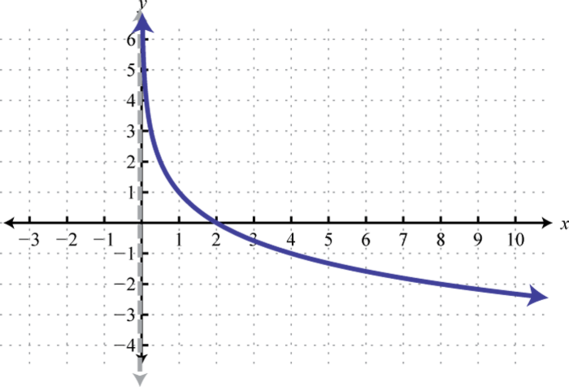$$f(x)=-\log _{2} x+1$$ 89. $$f(x)=\log _4(x)$$. Up 2$$f(x)=2+\log _4 x$$ 91. $$f(x)=\log_{1 / 3}(x)$$. Right 2, reflect over x-axis, up 1$$f(x)=1-\log _{1 / 3}(x-2)$$ 93. $$f(x)=\ln(x)$$. Reflect over x-axis, up 2$$f(x)=2-\ln x$$ 95. $$f(x)=\ln(x)$$. Reflect over y-axis$$f(x)=\ln(−x)$$ 97. $$f(x)=\log(x)$$. Left 2, reflect over x-axis$$f(x)=-\log (x+2)$$ 99. $$f(x)=\log(x)$$. Left 6, reflect over y-axis, horizontally shrunk by a factor of 1/3, up 1$$f(x)=\log (6-3x)+1$$ 103. $$f(x)=\log(x)$$. Vertically stretched by a factor of 2$$f\left(x\right)=2\log \left(x\right)$$

### I: Find the Equation for the Graph of a Logarithmic Function (no stretching)

Exercise $$\PageIndex{I}$$: Find the Equation for the Graph of a Logarithmic Function

$$\bigstar$$ For the following exercises, write a logarithmic equation corresponding to the graph shown.

 105.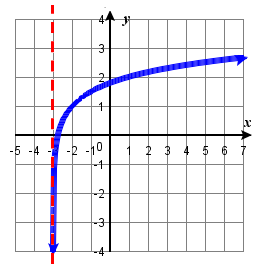106.107.  ($$(7,-3)$$ is on the graph).108.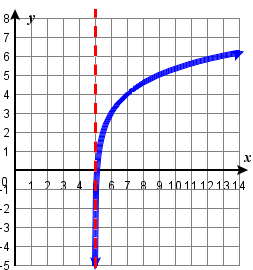$$\bigstar$$ For the following exercises, write a logarithmic equation corresponding to the graph shown.

 109.110.111.$$\bigstar$$ For the following exercises, write a logarithmic equation corresponding to the graph shown.

 113.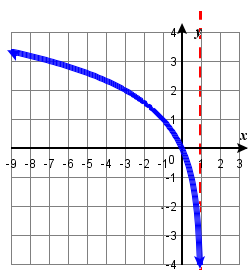114.105. $$f(x)=\log _4(x+3)+1$$  107. $$f(x)=\log _5(x-2)-4$$ 109. $$y = -\log_2 (x)$$ 111. $$y = -\log_2 (x+2)$$ 113. $$f(x)=\log _2(-(x-1))$$

### I: Find the Equation for the Graph of a Logarithmic Function (vertical stretching, no vertical shifting)

Exercise $$\PageIndex{I}$$: Find the Equation for the Graph of a Logarithmic Function

$$\bigstar$$ Write a logarithmic equation corresponding to the graph shown.

 120.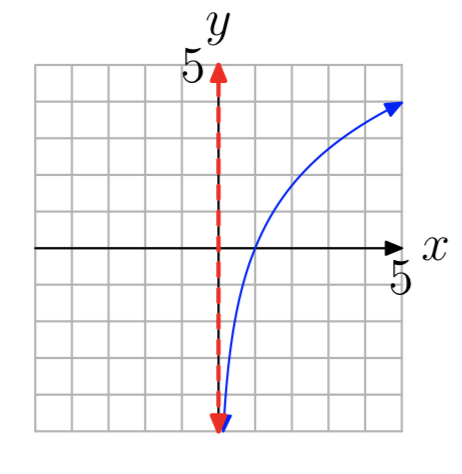121.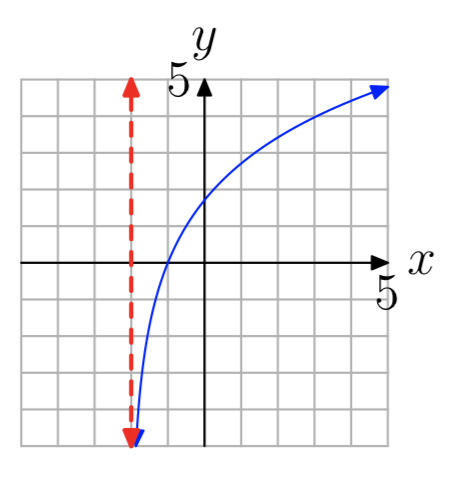122.123.124.125.$$\bigstar$$ Write a logarithmic equation corresponding to the graph shown.

 129. $$(-1,-3)$$ is on the graph130.131. $$(-6,2)$$ is on the graph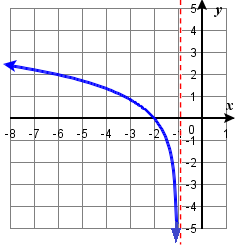132.$$\bigstar$$ Write a logarithmic equation corresponding to the graph shown.

 135.136.121. $$y = 4 \log_5 (x+2)$$ 123. $$f(x)=3\log _4(x+2)$$ 125. $$y = 3 \log_4 (x + 2)$$ 129. $$y = -3\log _3(x+4)$$ 131. $$y = 2\log _5(-x-1)$$ 135. $$y = -2\log_5 (-(x - 5))$$
$$\star$$